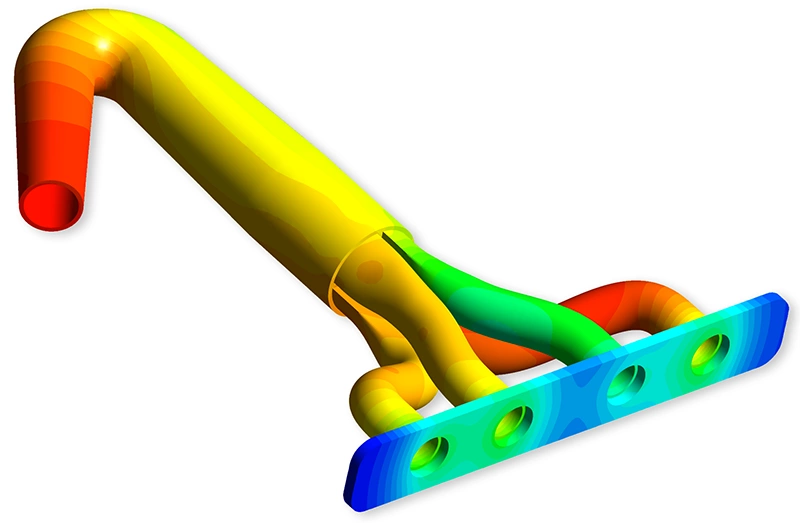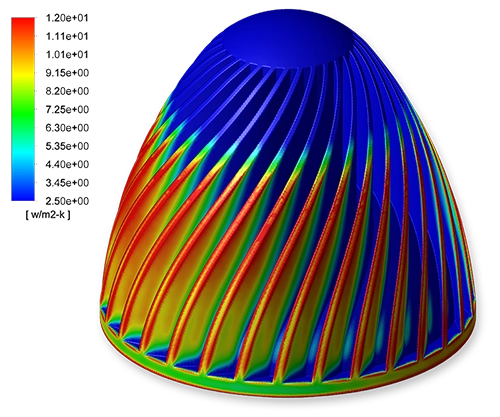# Heat Transfer Analysis

Heat Transfer Analysis has become a major aspect in many modern design processes. Most fluid flows, materials, parts and structures have temperature dependent characteristics that have an impact on the strength, performance and temperature level. Tools like Finite Element Analysis (FEA) and Computational Fluid Dynamics (CFD) can provide important information which are hard to obtain with analytical calculations or physical measurements.

## Thermal analysis

Thermal simulations are used for research & development, quality control and failure analysis, but also for regular design. Parts which are generating heat or are subject to external heat sources, like batteries for electric vehicles, electronic components on PCB's or solar boilers, all have benefit in decent research in efficient heat dissipation and temperature management.

Heat transfer analysis also contains the analysis of fluid flows and the heat transfer in fluids and between fluids and solids. Those analyses are often used for assessing chemical and pharmaceutical processes and for the development of innovative renewable-energy projects.

Two typical tools are used for thermal analysis: Finite Element Analysis (FEA) and Computational Fluid Dynamics (CFD).Heat transfer analysis of an electronic box done with CFD. The streamlines through the device are shown, as well as the temperatures of the individual electronic components.

## Heat transfer analysis with FEA

Thermal analysis with FEA allows for solving the heat transfer in or between solids. Heat transfer mechanisms such as convection, conduction and thermal radiation can be calculated. Convection and thermal radiation however, are explicitly defined by the analyst, and are not calculated by the FEA software.

Heat transfer analysis with FEA is mainly used in cases where the heat convection coefficient can be assumed constant over the part's surface or when the coefficient is known with sufficient accuracy.

Heat Transfer analysis with FEA also allows to calculate thermal expansion and structural stresses due to thermal gradients in the part.The temperature plot in a manifold due to the flow of hot exhaust gasses through the manifold. The temperatures in thed flow were calculated with CFD, while the temperatures in the manifold were calculated with FEA, based on the CFD results.

## Heat transfer analysis with CFD

When Computational Fluid Dynamics is used to perform a thermal analysis, the heat transfer between a fluid and a solid can be calculated, as well as the convection within the surrounding fluid itself.

The convection between a fluid and one or more solid parts is calculated by the software and can take away the uncertainty around the variation of the heat transfer coefficient by performing an accurate analysis of the fluid flow. The local velocities along the part's surface and the occurrence of turbulence in combination with temperature dependent properties of the fluid all have an impact on the convective heat transfer and are calculated with CFD with high precision.Display of the heat transfer coefficient h, calculated with CFD. The heat transfer coefficient is clearly not homogeneous along the surface of the heat sink. The red zones have a higher coefficient than the blue zones and are therefore more efficient in dissipating heat. This level of detail of convection heat transfer cannot be achieved with FEA.

## Fluid-Structure Interaction (FSI)

Structural deformations and stresses due to temperature variations in a component can be calculated with FEA. The temperature values can come from a heat transfer analysis done with FEA, or from a CFD analysis. In the latter case, the CFD and FEA solvers are coupled and temperature (and fluid pressure) results are shared. This coupled analysis is called a Fluid Structure Interaction (FSI) analysis.Streamlines through a shell-and-tube heat exchanger. The streamlines are shown for the flow through both the shell and through the tube side of the heat exchanger. The streamlines are colored with the temperature of the fluid.